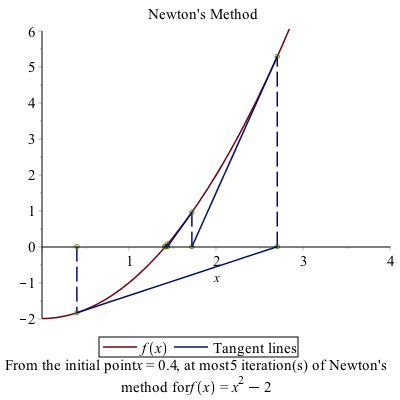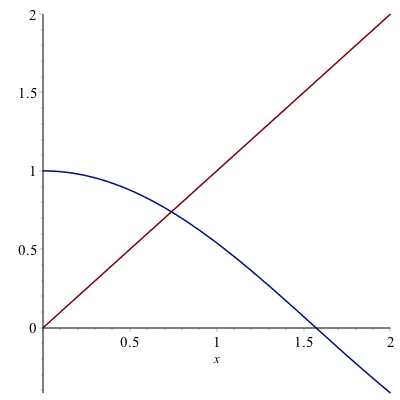# Chapter 12: Recursive Iteration, Rootfinding and Newton’s Method

### Rootfinding and the Bisection method

Recall that we encountered the Bisection method in Chapter 5. This was another way to do rootfinding. If we reenter the bisection procedure:

bisect := proc (f::procedure, interval::range,{eps:=10^(-4)})::numeric;
description "This procedure performs the bisection method on the function f with given range.  The parameter eps will determine  the halting condition."
local mid := .5*(lhs(interval)+rhs(interval));
local inter := interval;
if f(rhs(inter))*f(lhs(inter))>0 then
error "A root is not guaranteed to be in this interval.":
end if:
while rhs(inter)-lhs(inter) > eps do
if f(lhs(inter))*f(mid) < 0 then
inter := lhs(inter) .. mid
else
inter := mid .. rhs(inter)
end if;
mid := .5*(lhs(inter)+rhs(inter));
end do;
return mid:
end proc:

We can find roots, like $\sqrt{2}$ using this instead.

bisect(x->x^2-2,1..2)

### Exercise

Find the solution to $\cos x = x$ using the bisection method.

## Recursive Iterations

Earlier, we have seen a few cases of iteration, in particular recursive iteration. For example, the sequence:
$$a_{n} = \frac{1}{a_{n-1}+1}$$
with $a_0=1$ generates a sequence. In Chapter 10 we found the first few terms and then found the limit of this. Recall that if we define:

a:=n->1/(1+a(n-1))
a(0):=1

then seq(a(n),n=1..10) generates the first 10 terms or
$$1,\frac{1}{2},\frac{2}{3},\frac{3}{5},\frac{5}{8},{\frac {8}{13}},{\frac {13}{21}},{\frac {21}{34}},{\frac {34}{55}},{\frac {55}{89}},{\frac {89}{144}}$$

and as we discovered above, this sequence converges to the golden mean (or golden ratio)
$$\frac{1+\sqrt{5}}{2}$$

## Finding $\sqrt{a}$

Another useful iterative sequence is the following:
$$x_{n+1} = \frac{x_n^{2}+a}{2x_n}\qquad x_0=a$$

for a positive number $a$. We will show that this converges to $\sqrt{a}$. For example, if $a=2$, then

x:=n->(x(n)^{2}+2)/(2*x(n-1))
x(0):=2

(and again make sure that the $x_0$ is entered after the general formula.) If we find the first few terms of this we get:
$$\frac{3}{2},\frac{17}{12},\frac{577}{408},\frac{665857}{470832},\frac{886731088897}{627013566048},\ldots$$

and then the fraction gets quite large because $x_0$ is an integer and Maple is trying to keep everything as exact numbers (so returns a rational). If instead, we use $x(0):=2.0$, the rerun the first few terms:
$$2.0,1.500000000,1.416666666,1.414215686,1.414213562, \ldots$$

### Exercise.

Use the above to find $\sqrt{5}$.

## Newton’s Method

(First, before trying any of these, you’ll probably want to unassign('x') to get everything to work correctly.)

The above formula is a specific case of Newton’s method for finding roots. Here’s a geometric view of Newton’s method.

We intend to find the point $x^{\star}$ such that $f(x)=0$. We don’t know how to find $x^{\star}$, but have an approximate solution, call it $x_0$. Draw the tangent line to the curve $y=f(x)$ at $x_0$. The following plot is an example with $f(x)=x^{2}-2$ and $x_0=0.4$:The point on the left is $x_0=0.4$ and the blue line is the tangent line there. Instead of using the function, we use the tangent line, which is easy to solve. That value is $x_1=2.7$. At this point (no pun intended), we find the tangent line at $x_1$ and find where it crosses the $x$-axis.

This process continues indefinitely and (for this example), it converges to $\sqrt{2}$.

### Why this works

Recall that the tangent line to the curve $y=f(x)$ at $x=a$ is
$$y=f'(a)(x-a)+f(a)$$
and if we set $y=0$ in the tangent line because we are seeking the root (where the function is 0)
$$0=f'(a)(x-a)+f(a)$$
and then we solve for $x$. (Either by hand or let Maple do it).
$$x=a+\frac{f(a)}{f'(a)}$$

Next, if we let $a=x_0$, our initial point and $x$ be $x_1$, the next point:
$$x_1=x_0+\frac{f(x_0)}{f'(x_0)}$$

or in general:
$$x_{n+1}=x_n+\frac{f(x_n)}{f'(x_n)}$$

and this last equation is called Newton’s rootfinding method (or just Newton’s method)

### Example

Use Newton’s method to find the solution to $\cos x = x$.

If we let $f(x)=x-\cos x$ and perform Newton’s method. Since, $f'(x)=1+\sin x$, this means that
$$x_{n+1} = x_n - \frac{x_n-\cos x_n}{1+\sin x_n}$$
and let’s use $x_0=0$ as an initial guess.

In Maple, if we enter:

x:=n->x(n-1)-(x(n-1)-cos(x(n-1)))/(1+sin(x(n-1)))
x(0):=0.0

and then seq(x(n),n=0..5) results in
$$0., 1.000000000, 0.7503638679, 0.7391128909, 0.7390851334, 0.7390851332$$

As you can see from the sequence, the last two numbers are the same for the first 9 digits.

And looking at a plot of $x$ and $\cos x$,The solution looks like about 0.73.

### Exercise

There are two $x$ values such that $1-x^{2}=\sin x$. Find the solution that satisfies $x\lt 0$. Plot the two function to see if your answer is reasonable.

### Using the built-in NewtonsMethod command

There is a command called NewtonsMethod in the Student[Calculus1] package. Look at the Help Browser for the command and explore the options. Reproduce the problems above.